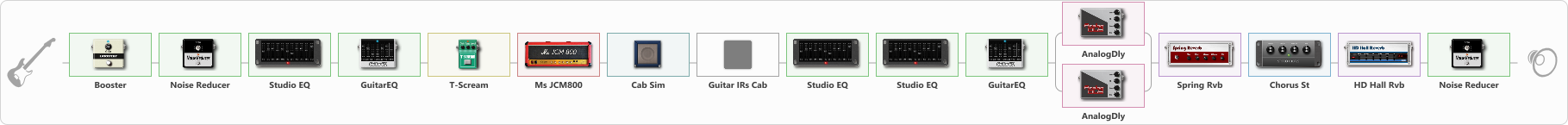# Alexi Laiho tribute

Discussion in 'ToneLib-GFX presets' started by truss van halen, Jan 18, 2021.

1. ### truss van halenWell-Known Member

Alexi Laiho tribute

Preset name: Alexi Laiho tribute

Effects chain:Effect: "Booster" (Dynamics / Filter), active - "yes"
{
"Gain" = 48
}

Effect: "Noise Reducer" (Dynamics / Filter), active - "yes"
{
"Sens" = 100
"Mode" = Hard
}

Effect: "Studio EQ" (Dynamics / Filter), active - "yes"
{
"31 Hz" = 0
"62 Hz" = 0
"125 Hz" = 0
"250 Hz" = 0
"500 Hz" = 0
"1 kHz" = 4
"2 kHz" = 3
"4 kHz" = 6
"8 kHz" = -3
"16 kHz" = 0
"Level (dB)" = 0
}

Effect: "GuitarEQ" (Dynamics / Filter), active - "yes"
{
"160 Hz" = 0
"400 Hz" = 0
"800 Hz" = 0
"1.6 kHz" = 0
"3.2 kHz" = 0
"6.4 kHz" = 7
"12 kHz" = 0
"Level (dB)" = 5
}

Effect: "T-Scream" (Overdrive / Distortion), active - "yes"
{
"Drive" = 56
"Tone" = 51
"Level" = 79
}

Effect: "Ms JCM800" (Amp simulators), active - "yes"
{
"Gain" = 58
"Bass" = 65
"Middle" = 46
"Treble" = 69
"Presence" = 30
"Master" = 52
"Level (dB)" = 4
}

Effect: "Cab Sim" (Cabinets), active - "yes"
{
"Model" = 4x12" Greenback
"Level (dB)" = 0
}

Effect: "Guitar IRs Cab" (Cabinets), active - "no"
{
"Model" = Mesa Rectifier (4x12")
"Mic Position" = Middle
"Mic Distance" = Middle
"Low Cut (Hz)" = 78
"Hi Cut (kHz)" = 20.0
"Mix" = 25
"Level (dB)" = -2
}

Effect: "Studio EQ" (Dynamics / Filter), active - "yes"
{
"31 Hz" = 3
"62 Hz" = 0
"125 Hz" = 0
"250 Hz" = 0
"500 Hz" = 1
"1 kHz" = -2
"2 kHz" = 0
"4 kHz" = 3
"8 kHz" = 4
"16 kHz" = 5
"Level (dB)" = 2
}

Effect: "Studio EQ" (Dynamics / Filter), active - "yes"
{
"31 Hz" = -2
"62 Hz" = -3
"125 Hz" = 0
"250 Hz" = 0
"500 Hz" = 0
"1 kHz" = 0
"2 kHz" = 0
"4 kHz" = -4
"8 kHz" = -4
"16 kHz" = -13
"Level (dB)" = -1
}

Effect: "GuitarEQ" (Dynamics / Filter), active - "yes"
{
"160 Hz" = -2
"400 Hz" = -2
"800 Hz" = 2
"1.6 kHz" = 0
"3.2 kHz" = -5
"6.4 kHz" = -6
"12 kHz" = -8
"Level (dB)" = 1
}

Effect: "Splitter" (Dynamics / Filter), active - "yes"
{
"A-Bypass" = Off
"A-Pan" = -49
"A-Level" = 55
"B-Bypass" = Off
"B-Pan" = 46
"B-Level" = 55

'A' branch:
{

Effect: "AnalogDly" (Delay), active - "yes"
{
"Time" = 452
"Feedback" = 52
"Tone" = 61
"Mix" = 57
}
}
'B' branch:
{

Effect: "AnalogDly" (Delay), active - "yes"
{
"Time" = 812
"Feedback" = 59
"Tone" = 56
"Mix" = 57
}
}
}

Effect: "Spring Rvb" (Reverberation), active - "yes"
{
"Time" = 3.5
"PreDelay" = 0
"LoDamp" = 16
"HiDamp" = 26
"Mix" = 47
}

Effect: "Chorus St" (Modulation / Sfx), active - "yes"
{
"Speed" = 1.9
"Depth" = 0
"Center" = 4.4
"Mix" = 40
}

Effect: "HD Hall Rvb" (Reverberation), active - "yes"
{
"Time" = 3.2
"PreLPF" = 44
"PreDelay" = 96
"HiDamp" = 27
"LoGain" = -3.3
"Mix" = 48
}

Effect: "Noise Reducer" (Dynamics / Filter), active - "yes"
{
"Sens" = 59
"Mode" = Soft
}

Note: You will need to download and install the ToneLib-GFX software to use the preset.

File size:
1.7 KB
Views:
3,557